# Dual Energy Tests (Part 2)

## September 30, 2015

This post focuses on when the dual energy formalism should have an effect. From Bryan et al., 2014 (B14): “It should be stressed that as long as the parameter $\eta_1$ is small enough the dual energy method will have no dynamical effect.”

Here, $\eta_1$ refers to the ratio that determines whether a given cell updates its internal energy using the conservative update vs. the non-conservative update. As given in B14, Equation 44:

For the following tests, we use the Teyssier 2015 (T15) nonconservative update (Equation 161), with a centered-difference estimate for the divergence of the velocity:

We are using the B14 switch described above to calculate the internal energy for a given cell. We are also using the B14 method to decide when to synchronize the internal energy for a cell with the total energy. This is done again according to a ratio of internal to total energy, but this ratio takes into account the maximum energy of nearby cells, as well:

Note that in the tests shown below, the bulk velocity is high enough that the total energy is synced for every cell each timestep. That is, the ratio of conservatively calculated internal energy to maximum nearby total energy is always much less than $\eta_2$.

The tests

Is the statement above regarding dynamical impact true for Cholla?

The tests shown below consist of a shock tube with periodic boundaries, to allow for high mach number bulk flow.

For the high mach number test, $v_L = v_R = 120$. The tests are run until $t = 0.8$, on a domain from -3 to 3.

Without Dual Energy

Figure 1: PPMP, exact solver, no dual energy, $v_{bulk} = 100 c_s$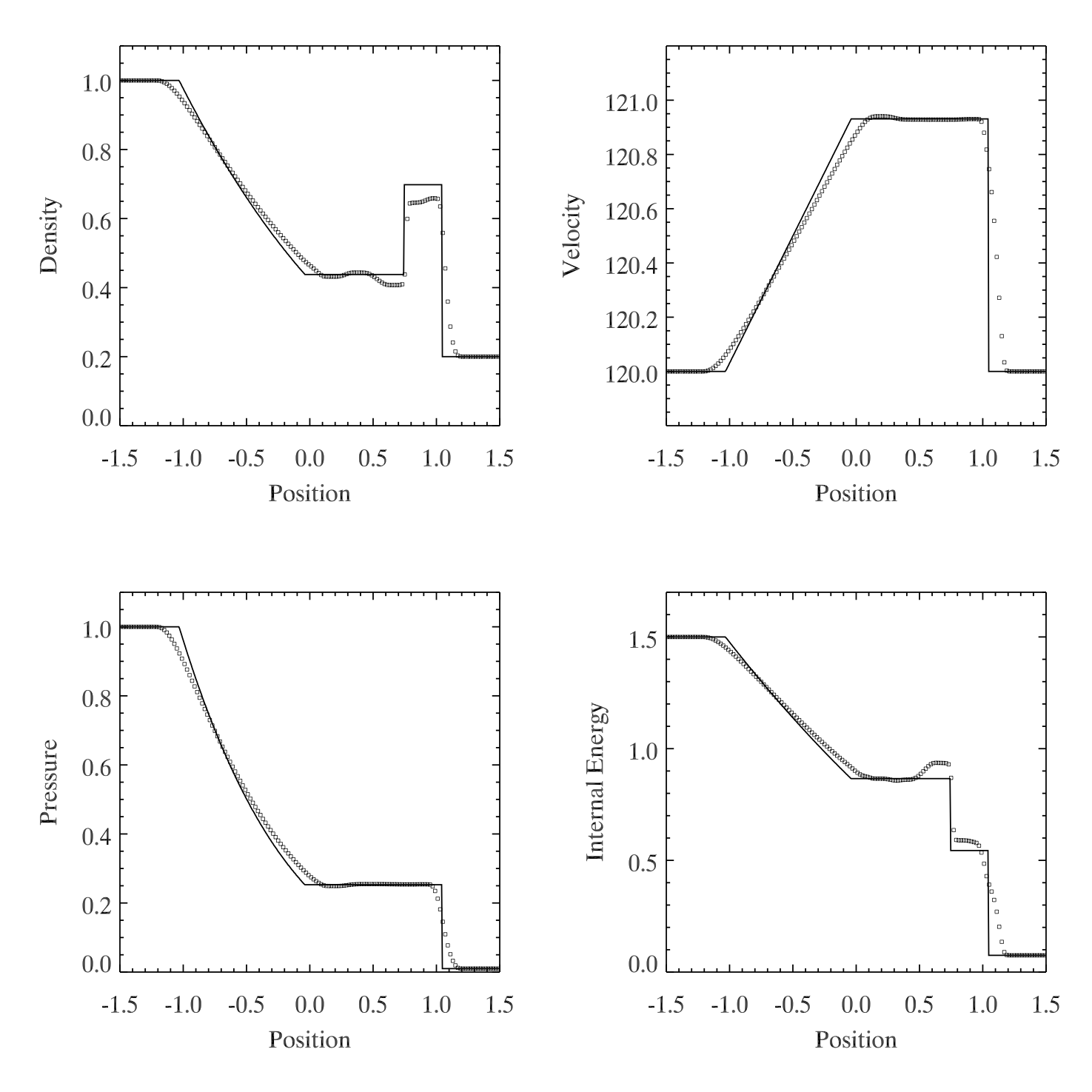With Dual Energy

Figure 2: Same as figure one but with dual energy, $\eta_1 = 10^{-6}$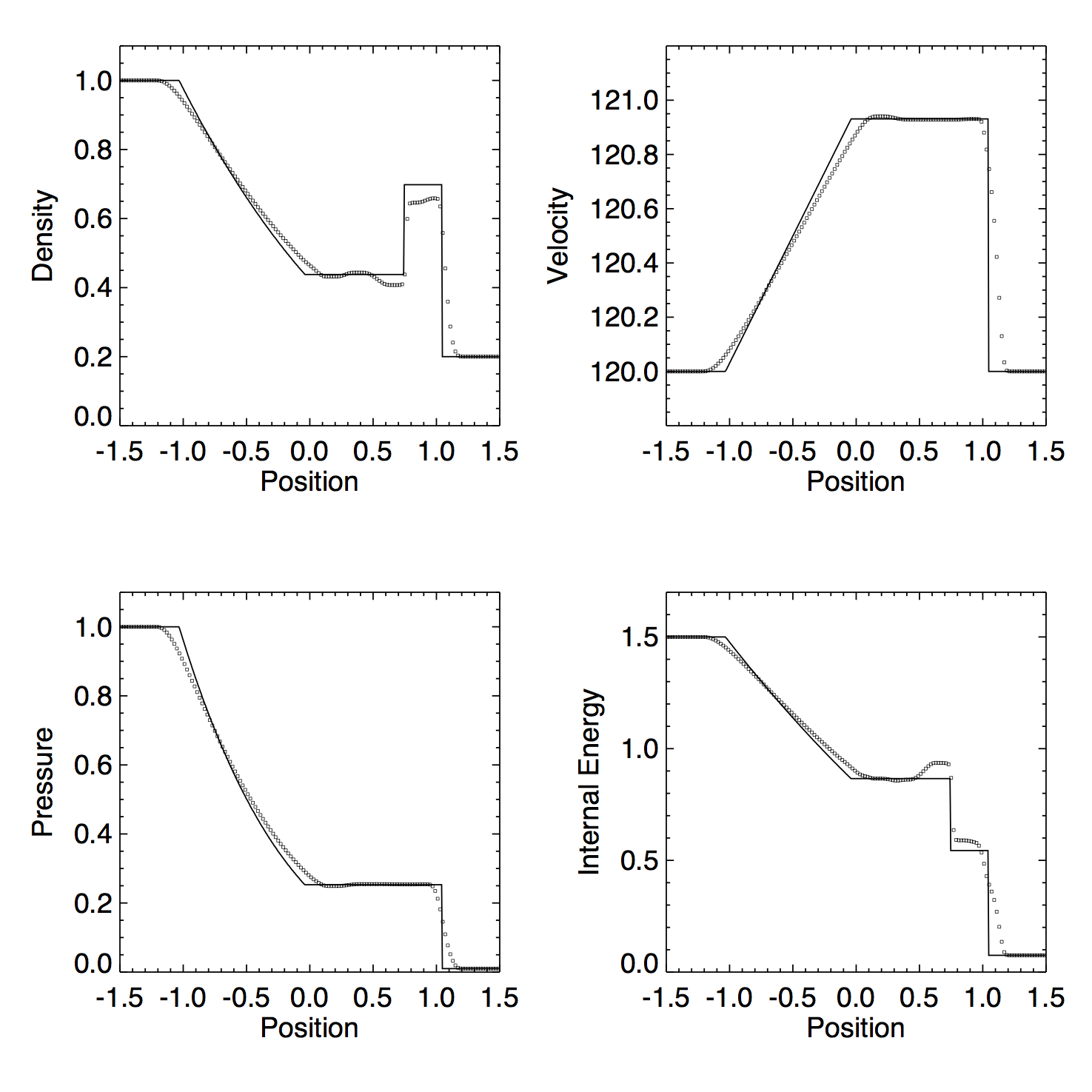As expected, the dual energy formalism has no effect in this case. Practically speaking, this is because the internal energy for each cell is reset to the conservatively calculated value at every timestep, NOT because the hydro update is unaffected by a non-conservatively calculated internal energy.

So what happens if we increase $\eta_1$?

With $\eta_1 = 10^{-5}$ one cell every so often (not every time step) chooses the non-conservative internal energy update. The non-conservative $e$ in these cases is always slightly larger than the conservatively calculated $e$. Nevertheless, there is not a discernable dynamic impact.

With $\eta_1 = 10^{-4}$ all cells to the right of the discontinuity (where $e = 0.075$ initially) are using the non-conservative update. As the test begins, the two energies are the same for the majority of these, because there is no energy flux or velocity gradient. For the cells within the shock, however, the two values differ. At first, the non-conservative update has higher internal energy values, but as the test continues the values are sometimes lower. It seems that the non-conservative internal energy values are suffering from osciallations. Perhaps the instability is a result of the centered-difference approximation?

After time $t = 0.1863830$ the conservatively calculated internal energy is occasionally negative. The test does run to completion without the non-conservative energy update producing negative pressures, but the results are a mess in the regions where the non-conservative update was used.

Figure 3: Same as Figure 1 but with dual energy, $\eta_1 = 10^{-4}$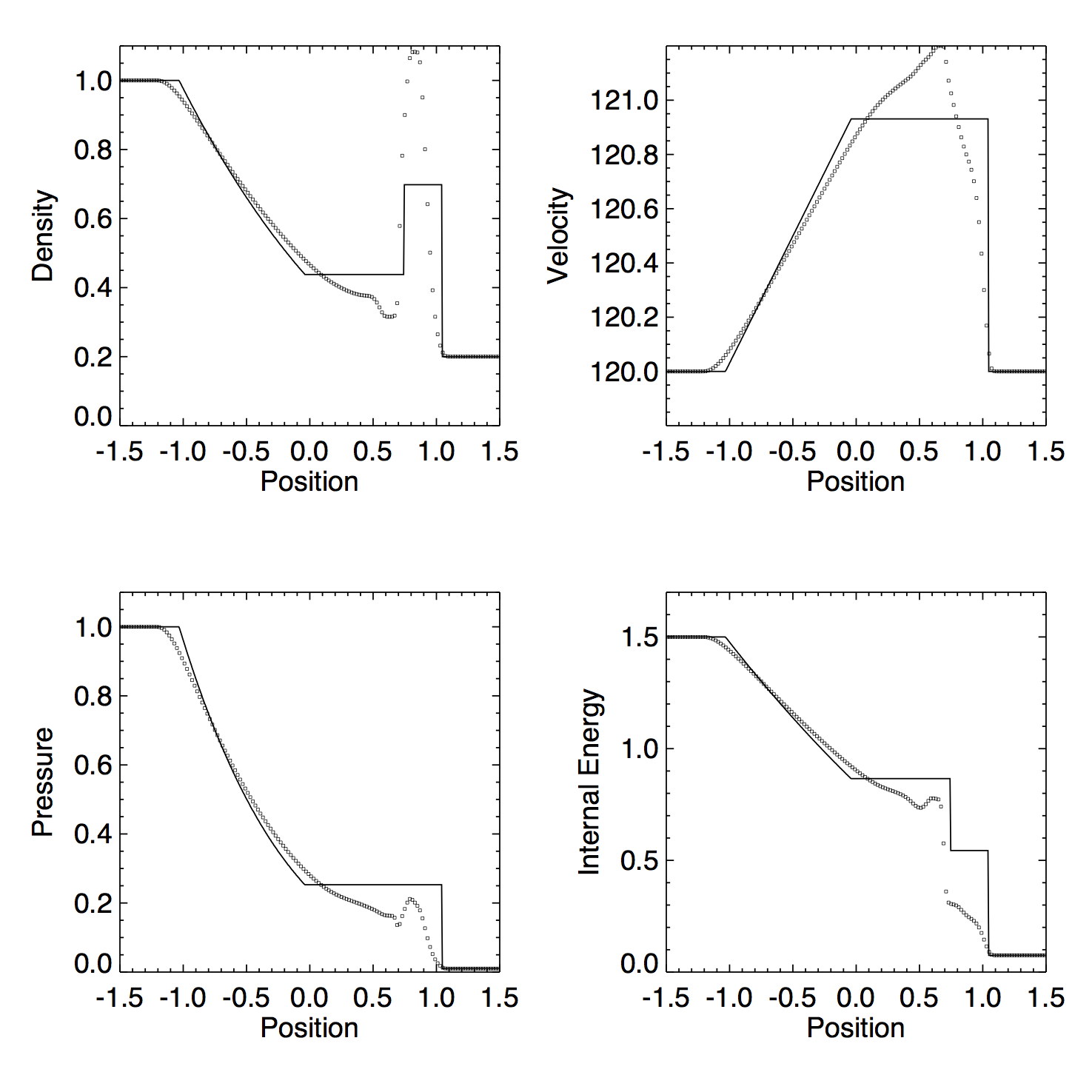With $\eta_1 = 10^{-3}$ (the value given in B14), all cells are using the non-conservative energy update, and the results are a bit better (but still inferior to the non dual energy case).

Figure 4: Same as Figure 1 but with dual energy, $\eta_1 = 10^{-3}$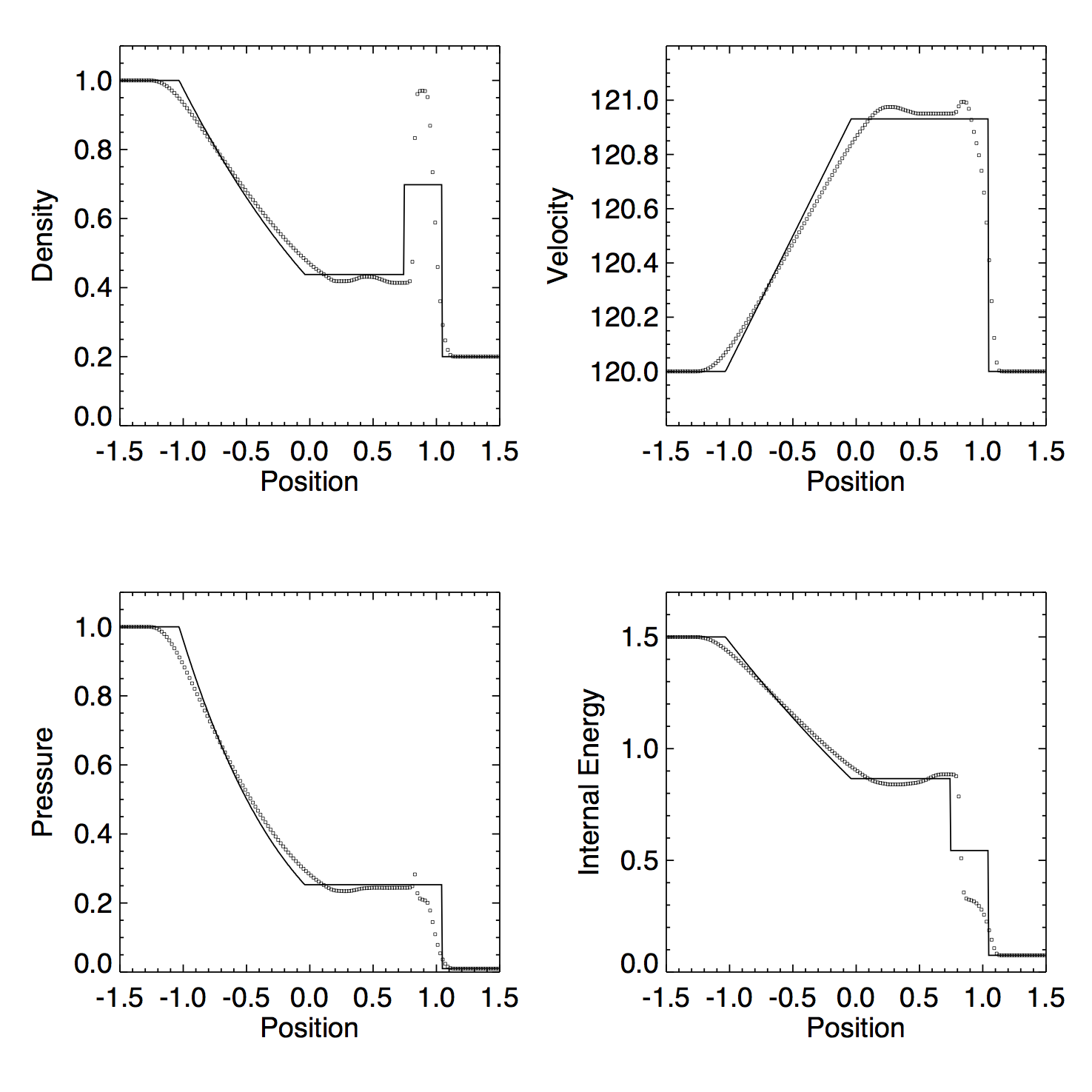Is it the way the divergence is approximated?

B14 have a slightly different way of estimating the $P \nabla \cdot \mathbf{v}$ term. They use the velocities at the interface returned by the Riemann solver to approximate the derivative. Does using this version help?

Figure 5: Same as Figure 4 but with the B14 internal energy update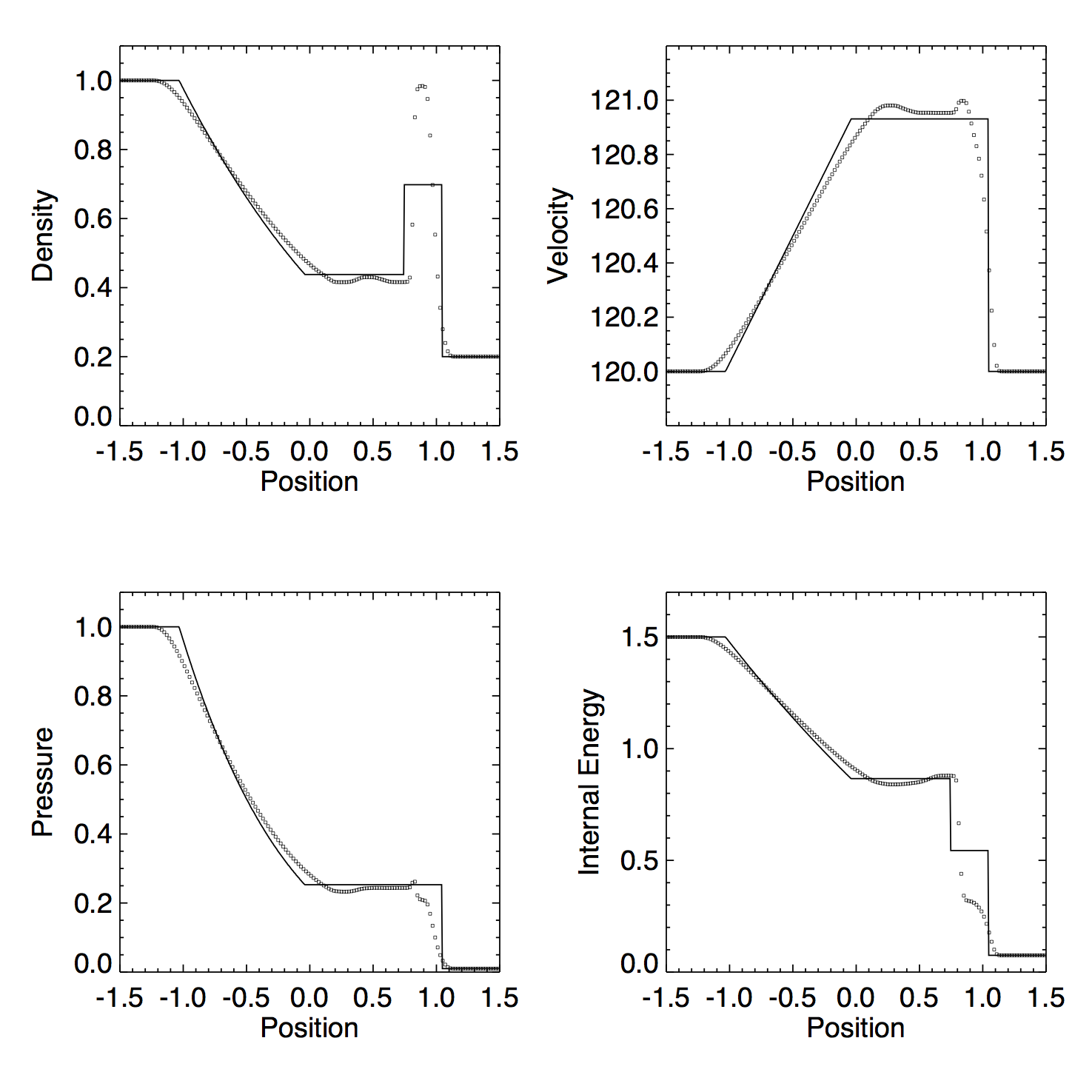The results are strikingly similar to Figure 4. Evidently the Pdv approximation is not the problem. Perhaps there is an issue somewhere else in the calculation. The next post will use a high mach advection problem to test this.